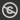Science, Maths & Technology
Free course

# Linear programming – the basic ideas## Course reviews

This free course examines the formulation and solution of small linear programming problems. Section 1 deals with the formulation of linear programming models, describing how mathematical models of suitable real-world problems can be constructed. Section 2 looks at graphical representations of two-dimensional models, considers some theoretical implications and examines the graphical solution of such models. Section 3 introduces the simplex method for solving linear programming models and Section 4 uses matrix notation to formalize the simplex method.

## Course learning outcomes

After studying this course, you should be able to:

• formulate a given simplified description of a suitable real-world problem as a linear programming model in general, standard and canonical forms
• sketch a graphical representation of a two-dimensional linear programming model given in general, standard or canonical form
• classify a two-dimensional linear programming model by the type of its solution
• solve a two-dimensional linear programming problem graphically
• use the simplex method to solve small linear programming models by hand, given a basic feasible point.

First Published: 12/03/2018

Updated: 11/07/2019

Skip Rate and Review